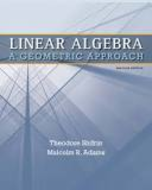Linear Algebra: A Geometric Approach - 2 Edition - Chapter 3.5 - Problem 4
Register Now

Join StudySoup

Get Full Access to Linear Algebra: A Geometric Approach - 2 Edition - Chapter 3.5 - Problem 49781429215213

# a. Show that in a graph with n nodes and n edges, there must be a loop. b. A graph is

Linear Algebra: A Geometric Approach | 2nd Edition

Problem 4

a. Show that in a graph with n nodes and n edges, there must be a loop. b. A graph is called a tree if it contains no loops. Show that if a graph is a tree with n nodes, then it has at most n 1 edges. (Thus, a tree with n nodes and n 1 edges is called a maximal tree.) c. Give an example of an incidence matrix A with dim N(A) > 1. Draw a picture of the graph corresponding to your matrix and explain.

Accepted Solution
Step-by-Step Solution:

Step 1 of 3

Let G be a graph with n nodes and n edges.

Since G is connected, it must have a spanning tree T with n vertices andedges.

Let us consider an arbitrary edgesuch thatwith endpoints u and v.

Since T is a tree, there is a unique pathbetween u and V.

The union ofandis a cycle.

Thus, it is proved that in a graph with n nodes and n edges, there must be a loop.

A graph is called a tree if it contains no loops.

###### Chapter 3.5, Problem 4 is Solved

Step 2 of 3

Step 3 of 3

Unlock Textbook Solution